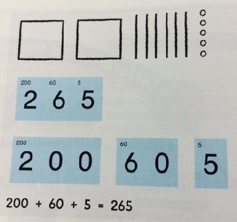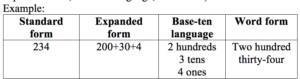Leaning the base ten system is like learning a new language for our children. Instead of letters there are the digits 0-9. Depending on the placement of the digit determines the value it represents. We group 10 ones to make 1 ten, 10 groups of ten to make 1 hundred and so on. This repeated pattern needs to be explored in many ways for children to build a deep and flexible understanding of the base ten system.For this reason, we explore many representations of a value. We begin with modeling objects and showing students how they can bundle or put together objects into groups of 10 and place it as 1 in the next greater place value. Then we use these representations to help describe the value in different forms. For example, a value can be written in standard form using digits and placing them in the correct place as in the number 147. Most adults have had experiences writing a number in word form when writing a check. Although many students have never had an opportunity to write a check, and it is uncertain if they ever will, it is still very important that they learn how to write a value in word form because if they know how to write a number they can read a number using proper place value language. Yet, the idea of expanded form or base-ten language are very foreign to many of us. So, why are our students learning this and why is it important?

1. Expanded form- is a way to write numbers by adding the value of its digits. Example: 900 + 50 + 4 = 954.

When looking at a value like 1,954 children often have difficulty understanding place value and the value of each digit. Many children when asked describe each digit as a value of ones. When looking at the example above a child may say that the digit 9 has a value of 9 rather than having a value of 900. When students expand each digit showing its value they gain a deeper understanding of the digit’s worth depending on its place within the number.2. Base ten language- is a way to use three digits of a three-digit number represent amounts of hundreds, tens, and ones; e.g., 706 equals 7 hundreds, 0 tens, and 6 ones.

The use of base ten language helps students to understand that a digit in a place value represents a grouping rather than just the value of the digit.

Investigating a value in so many ways sets the stage for better addition and subtraction strategies later in the year as well as multiplication and division strategies in third grade.